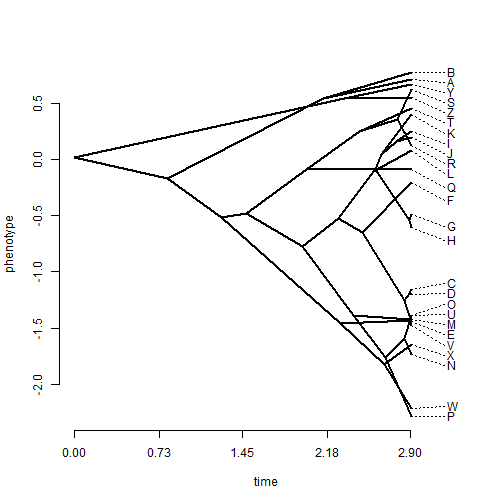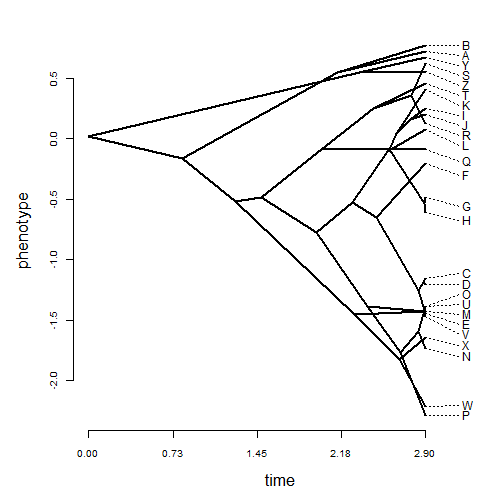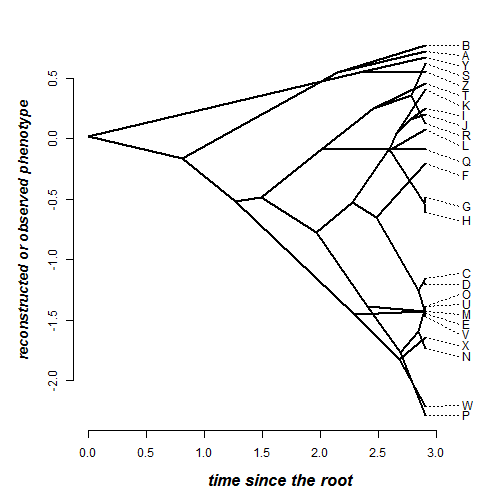## Tuesday, May 16, 2017

### Plotting a traitgram using `phenogram` with custom axis labels

This morning I received the following question about the phytools function `phenogram` for plotting a 'traitgram' (a projection of the tree into a one-dimensional phenotype space with time as the second dimension):

“I have a very quick question about constructing phenograms with ancestral states using phytools. I am trying to change the font size in the x and y labels as well as in the numerical values of both axes, but I can't. I have tried arguments such as '`cex.lab`', which usually work in other plots, without success. I was wondering if you could share with me the commands/arguments/script to change the font size in these labels and numerical values of these nice phenograms.”

There are multiple options to do this. Here are two that should work, depending on the flexibility desired.

Option 1: The `par` function.

``````library(phytools)
tree
``````
``````##
## Phylogenetic tree with 26 tips and 25 internal nodes.
##
## Tip labels:
##  A, B, C, D, E, F, ...
##
## Rooted; includes branch lengths.
``````
``````x
``````
``````##           A           B           C           D           E           F
##  0.71834934  0.77516371 -1.15527055 -1.20178011 -1.44344168 -0.20245893
##           G           H           I           J           K           L
## -0.48830753 -0.60784770  0.24882588  0.19768991  0.40526574  0.07893109
##           M           N           O           P           Q           R
## -1.42560735 -1.73332436 -1.38883804 -2.29045453 -0.08663248  0.12563454
##           S           T           U           V           W           X
##  0.62373799  0.45686318 -1.38973670 -1.47254717 -2.21400886 -1.64686733
##           Y           Z
##  0.67458737  0.55373959
``````
``````## first, our standard traitgram plot for reference:
phenogram(tree,x)
````````````## change the axis label size using par(cex.lab):
par(cex.lab=1.4,cex.axis=0.8,mar=c(5.1,5.1,2.1,1.1))
phenogram(tree,x)
``````Option 2: Plot without axes & add them in after.

``````par(xaxt="n",yaxt="n",mar=c(5.1,5.1,2.1,1.1))
phenogram(tree,x,xlab="",ylab="")
par(xaxt="s",yaxt="s",font.lab=4)
axis(1)
title(xlab="time since the root",cex.lab=1.4)
axis(2)
title(ylab="reconstructed or observed phenotype",cex.lab=1.2)
``````The second option is a bit more laborious, but obviously also offers considerably more flexibility.

That's it!

#### 1 comment:

1.Thank you very much! This was very useful indeed.
Gracias from Mexico City!

Note: due to the very large amount of spam, all comments are now automatically submitted for moderation.